Ex 11.1

Chapter 11 Class 10 Constructions [Deleted]
Serial order wise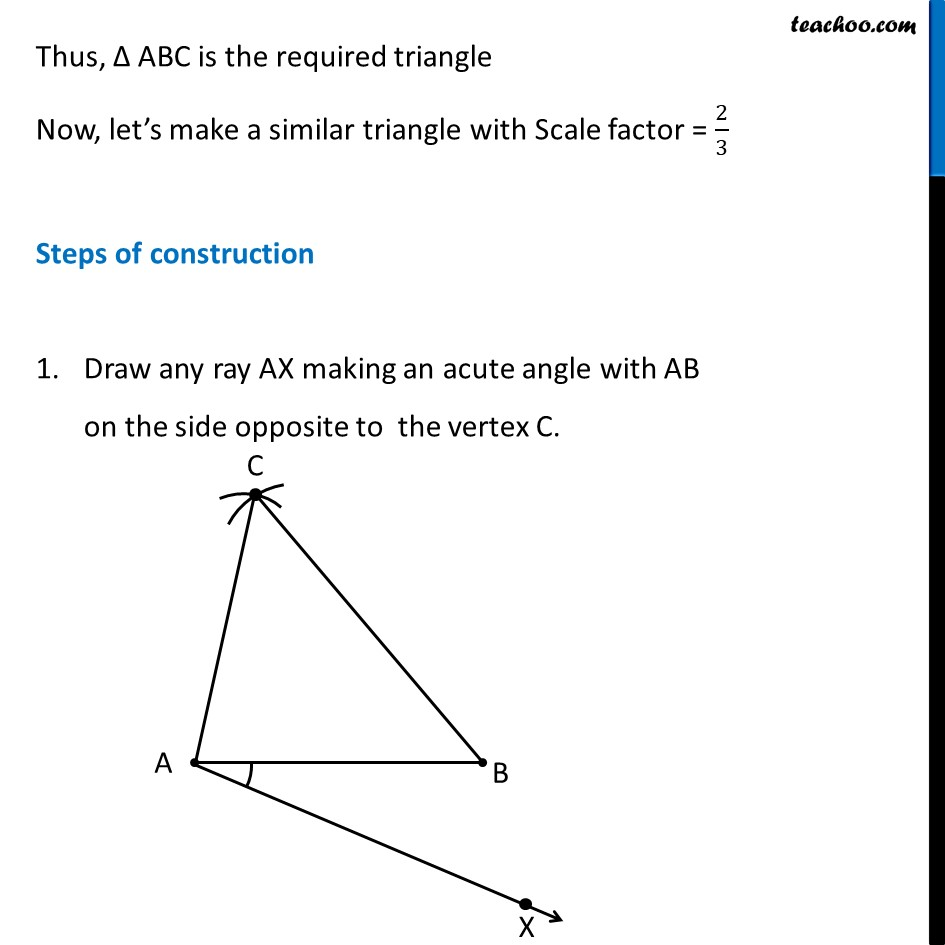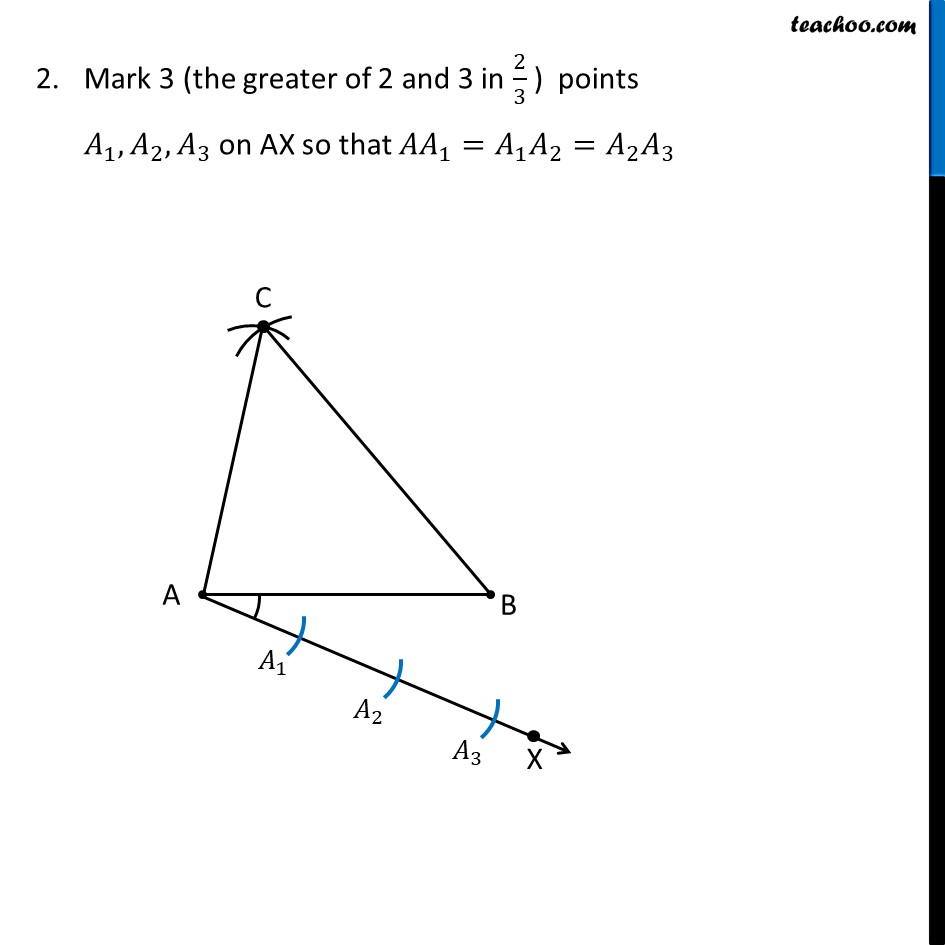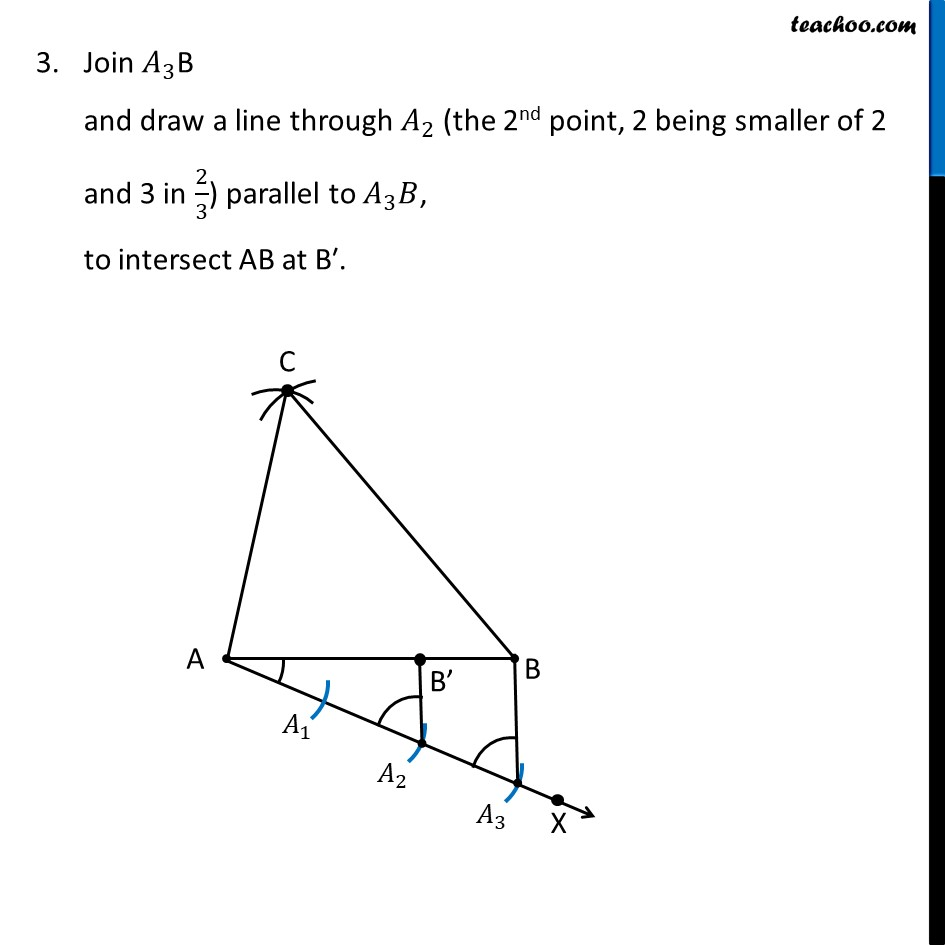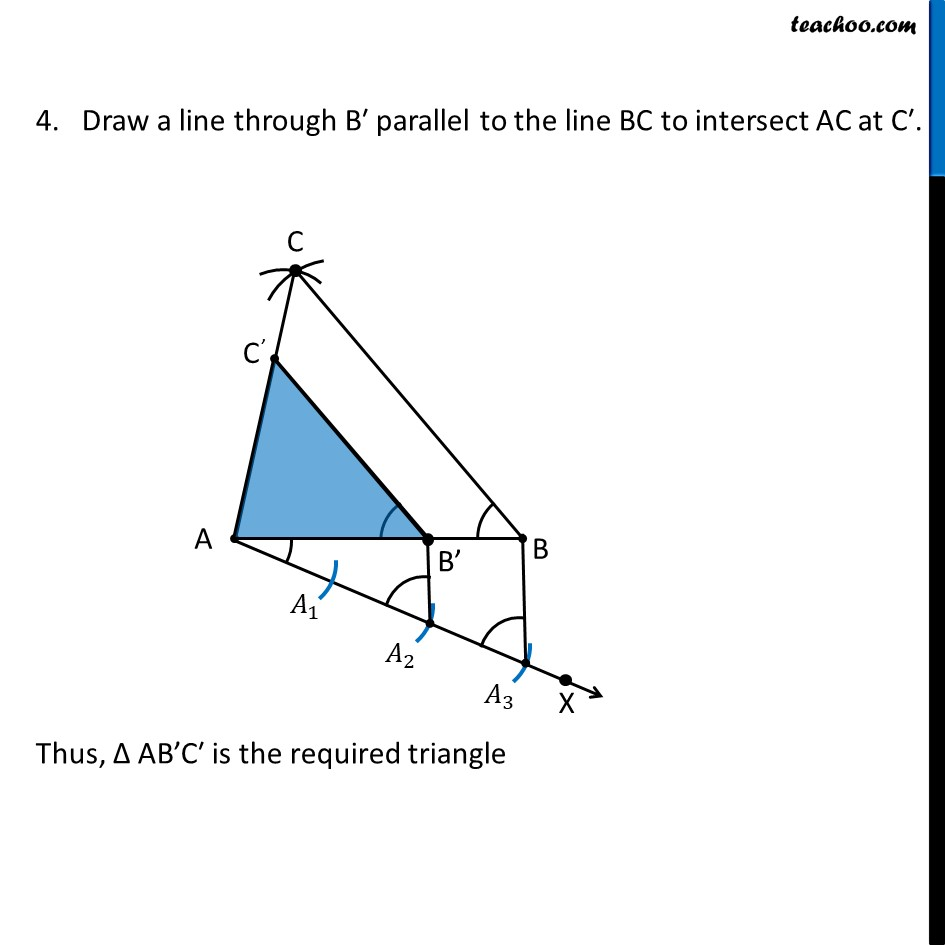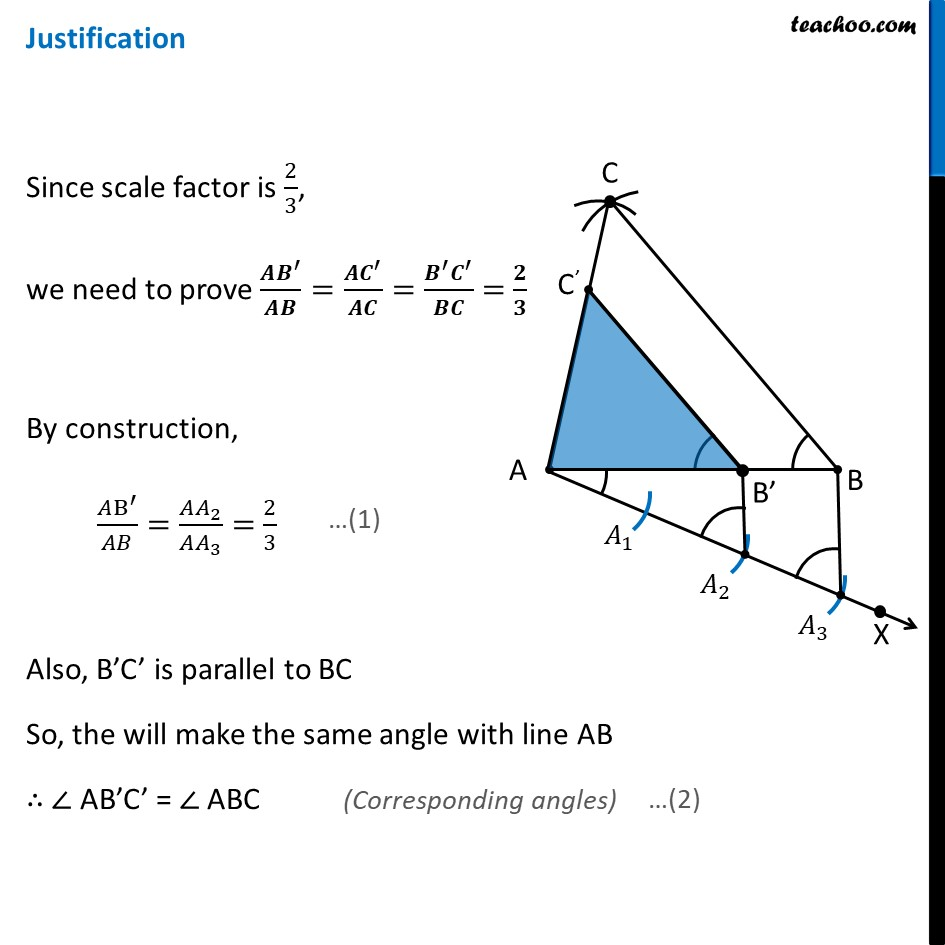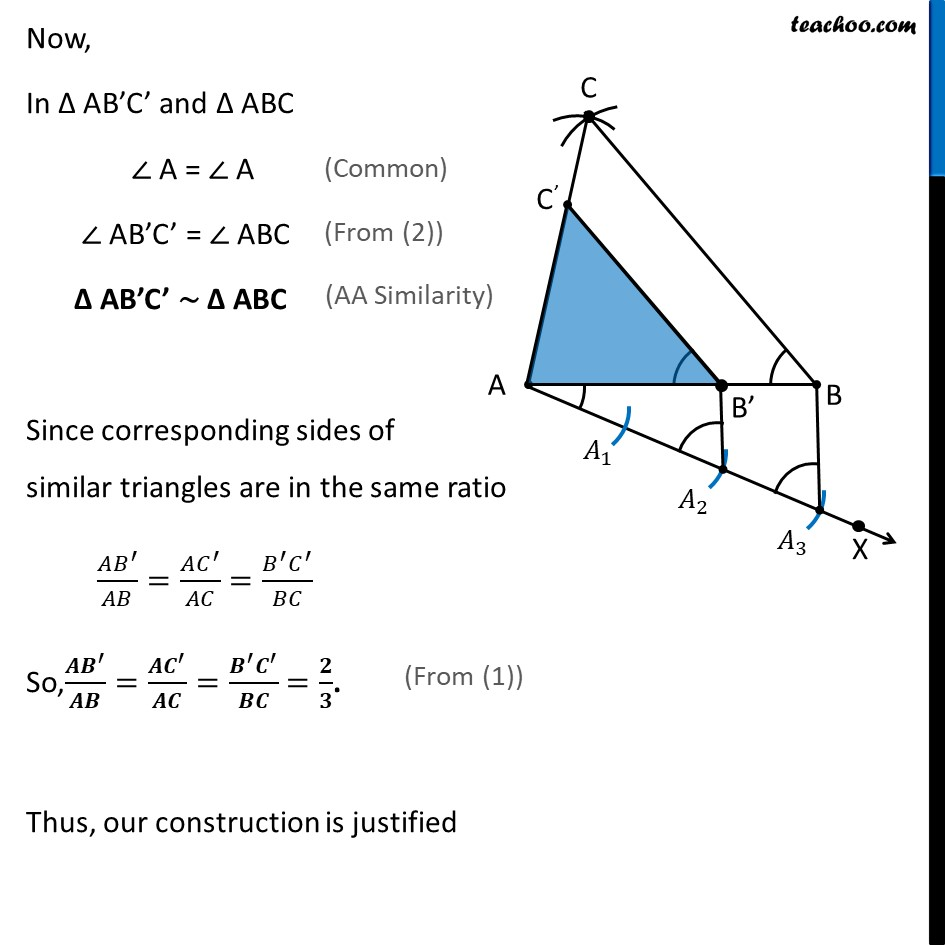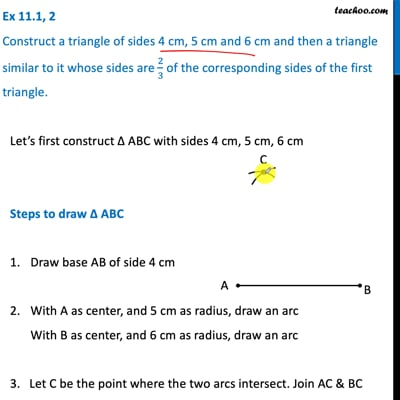This video is only available for Teachoo black users

Maths Crash Course - Live lectures + all videos + Real time Doubt solving!

### Transcript

Ex 11.1, 2 Construct a triangle of sides 4 cm, 5 cm and 6 cm and then a triangle similar to it whose sides are 2/3 of the corresponding sides of the first triangle. Let’s first construct Δ ABC with sides 4 cm, 5 cm, 6 cm Steps to draw Δ ABC Draw base AB of side 4 cm With A as center, and 5 cm as radius, draw an arc With B as center, and 6 cm as radius, draw an arc 3. Let C be the point where the two arcs intersect. Join AC & BC Thus, Δ ABC is the required triangle Now, let’s make a similar triangle with Scale factor = 2/3 Steps of construction Draw any ray AX making an acute angle with AB on the side opposite to the vertex C. Mark 3 (the greater of 2 and 3 in 2/3 ) points 𝐴_1, 𝐴_2, 𝐴_3 on AX so that 〖𝐴𝐴〗_1=𝐴_1 𝐴_2=𝐴_2 𝐴_3 Join 𝐴_3B and draw a line through 𝐴_2 (the 2nd point, 2 being smaller of 2 and 3 in 2/3) parallel to 𝐴_3 𝐵, to intersect AB at B′. 4. Draw a line through B′ parallel to the line BC to intersect AC at C′. Thus, Δ AB’C′ is the required triangle Justification Since scale factor is 2/3, we need to prove (𝑨𝑩^′)/𝑨𝑩=(𝑨𝑪^′)/𝑨𝑪=(𝑩^′ 𝑪^′)/𝑩𝑪 = 𝟐/𝟑 By construction, (𝐴B^′)/𝐴𝐵=(𝐴𝐴_2)/(𝐴𝐴_3 )= 2/3 Also, B’C’ is parallel to BC So, the will make the same angle with line AB ∴ ∠ AB’C’ = ∠ ABC (Corresponding angles) Now, In Δ AB’C’ and Δ ABC ∠ A = ∠ A (Common) ∠ AB’C’ = ∠ ABC (From (2)) Δ AB’C’ ∼ Δ ABC (AA Similarity) Since corresponding sides of similar triangles are in the same ratio (𝐴𝐵^′)/𝐴𝐵=(𝐴𝐶^′)/𝐴𝐶=(𝐵^′ 𝐶^′)/𝐵𝐶 So,(𝑨𝑩^′)/𝑨𝑩=(𝑨𝑪^′)/𝑨𝑪=(𝑩^′ 𝑪^′)/𝑩𝑪 =𝟐/𝟑. (From (1)) Thus, our construction is justified